Technical Information Site of Power Supply Design

2018.11.08 AC/DC

# Selecting Critical Components: Switch Setting Resistors for Overload Protection Points

Design Example of Isolated Quasi-Resonant Converters Using SiC MOSFET

This time, we will calculate resistance values related to settings for an overload protection correction function, which is a function specific to the power supply IC used in this design.

Selecting Critical Components :　The R20 Switch Setting Resistor for an Overload Protection Point

We begin by confirming the position on the circuit diagram of the resistor R20 to set the switch at an overload protection point. This circuit diagram is an excerpt of the relevant part of the overall diagram. If necessary, the entire circuit can be viewed here.

The BD7682F power supply IC used in this design has a function for correcting the overload protection point with respect to fluctuations in input voltage.

When the input voltage rises, if the overcurrent limit is fixed, there is a simple increase in the power loss. This correction function reduces power losses by lowering the current limit level when the input voltage rises above the setting value, to provide more secure protection during overloading.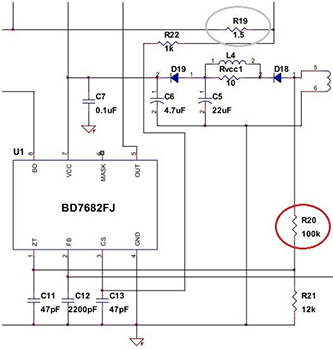The following is a calculation example. The circuit design assumes a three-phase 380 VAC input voltage. The maximum value of a three-phase 380 VAC input is √2×380 VAC = 537 VAC. Adding a margin of about 50%, the switching voltage is set to DC 800 V.

In the equation, Izt is the current from the IC flowing in the VCC windings Nd of the transformer when the switch is turned on. If Izt exceeds 1 mA, the overcurrent limit level is lowered, and the overload protection point is lowered.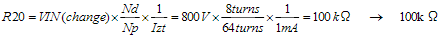Next, after the overload protection point has been switched, whether the rated load can be handled is determined. When the overload protection point is switched, Vcs = 1.0 V becomes 0.70 V. This means that the overcurrent limit is 0.7 times as large. The different parameters are calculated for these conditions.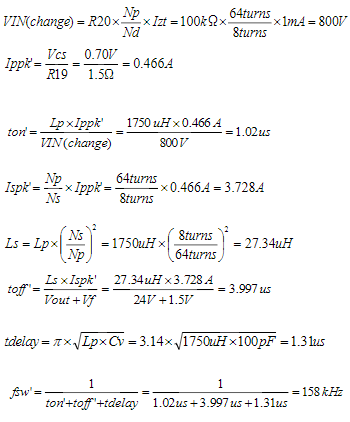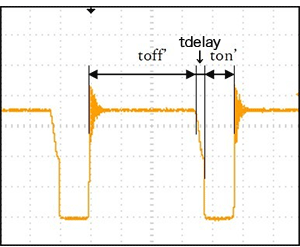If the transformer conversion efficiency is η = 0.85, then the output power after overload switching can be calculated using the following equation.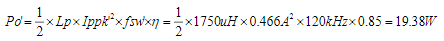In this equation, fsw' is 120 kHz rather than the calculation value of 158 kHz because the maximum switching frequency of the power supply IC is 120 kHz.

As indicated by the calculation result, for input voltages of 800 VDC or higher, the output voltage after the overload point has changed is limited to 19.38 W. Below are shown, for reference, measured values for the design example circuit. Overload protection points should be confirmed not only through calculations, but also with products incorporated into circuits.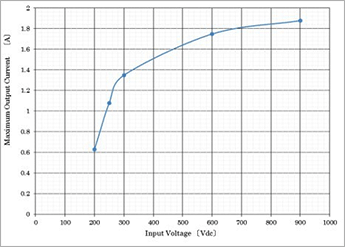Measured values using a design example circuit for overcurrent detection (reference)

#### Key Points:

・An overload protection correction function of this IC reduces power losses by lowering the current limit level when the input voltage rises above a setting value, to provide more reliable protection during overloading.

・The switching voltage is set through the resistance value of the resistor R20, calculated according to the equation provided.